# Scala安装与语言基础语法笔记

1. 右击我的电脑-> 点击”属性”->点击“高级系统设置”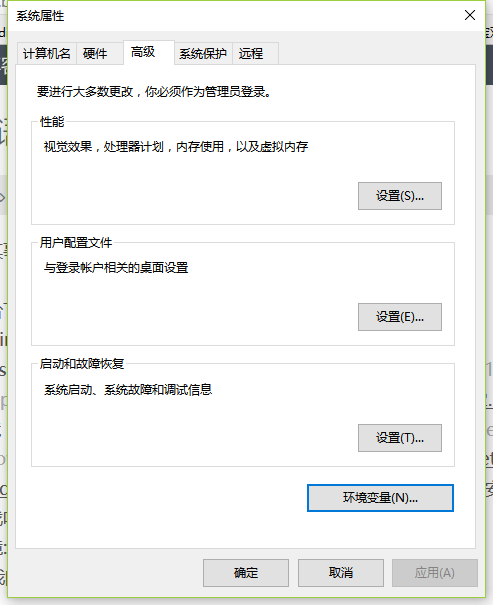2. 点击“环境变量”

ps:这里的添加的地址都是你所安装jdk scala的本地磁盘地址

3. 运行测试 判断是否安装成功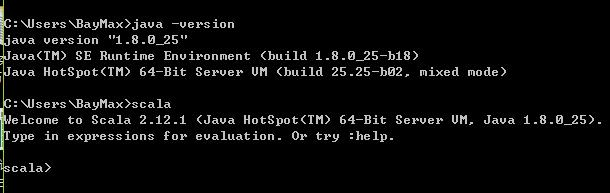IDE工具用的是IDEA的社区版本，下载地址：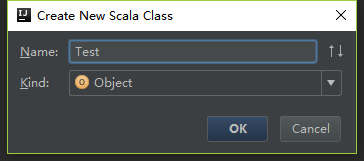Scala基础语法

Boolean类型Boolean

  val x = 0
var y = 1
y = 2
// 给常量赋值会出现编译错误
// x = 3
// 显式指定变量类型
val x1: Int = 0
var y1: Int = 0

if条件表达式
if/else语法结构和java一样，不过在scala中if/esle表达式有值，这个值就是if或else中最后一行语句返回的值。例如

object Test02 {
def main(args: Array[String]): Unit = {
val age=10
println(if(age>12)true else false)
}
//result : false

object Test02 {
def main(args: Array[String]): Unit = {
val age=20
val isadult = if (age>10) true else false
}
}
//result: true

object Test02 {
def main(args: Array[String]): Unit = {
val age=20
}
}
//result: true

if表达式的类型推断： scala中每个表达式都有一个类型，而if和else的值类型可能不同，此时会自动推断，取公共父类型。例如：

object Test02 {
def main(args: Array[String]): Unit = {
val age=20

println(if(age>10) "adult" else 0)
}
}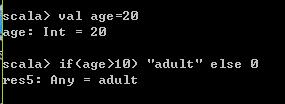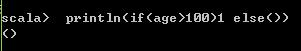scala类型图：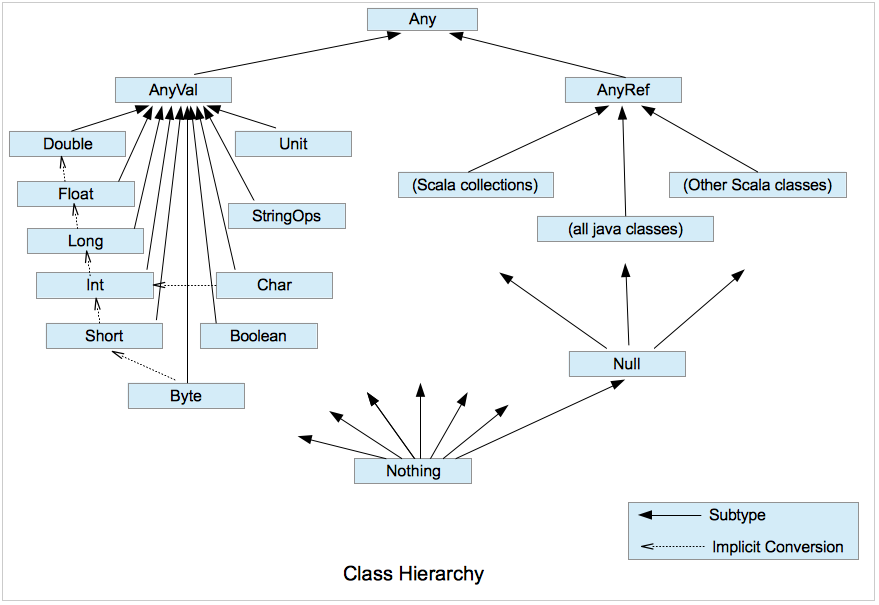var a,b,c=10;if(a>5){b=b+1;c=c+1} ;printf("%d,%d,%d",a,b,c)
//result  10,11 ,11

   var a,b,c=10
val res = if(a>5){ //res 为if语句最后一行返回值a+b+c
a+=1
b+=1
a+b+c
}
println(res)
}
//result: 32

scala拥有和java一样的while循环，没有for(初始化变量；边界条件；更新变量)循环，如果是这样的循环，则可以使用while循环或for语句实现(这个for语句与java中的for语句时有差别的，使用了一个类似R中的提取符号<-)
while循环：

  var a=5
while (a>0){
a=a-1 //注意 scala中并没有像java中的a-- 或者a++操作
print(a+" ")
}
//result:4 3 2 1 0 

do-while循环

var a = 5
do  {
a = a - 1
print(a + " ")
} while (a > 0)
//result:4 3 2 1 0 

while循环显示做判断，再执行代码块，而do-while是先执行代码块，再进行判断。

1.使用Boolean类型变量

2.使用return发挥

3.使用Breaks对象中的break方法

Boolean跳出循环：

var flag=true
var a=5
while(flag){
a=a-1
println(a)
if(a==2) {
flag = false
}
}

return跳出循环：

for (i<-1 to 10){
println(i)
if(i==5) return
}

public void f(){
return;
}

break跳出循环：

import scala.util.control.Breaks._
/**
....
*/
breakable {
for (i <- 1 to 10) {
println(i)
if (i == 5) break()
}
}

for(i<- 0 to 5) print(i+" ") 

until语句：

for (i<- 1 until(5)) print(i+" ")
//result: 1 2 3 4 

if守卫(即输出带if的bool类型的判断语句，满足某种条件输出)

for (i<- 1 to 20 if(i%2==0)) print(i+" ")//打印出1到20之间能被2整除的数字
//result:2 4 6 8 10 12 14 16 18 20

for生成集合

print(for (i<- 1 to 10) yield(i))
//result:Vector(1, 2, 3, 4, 5, 6, 7, 8, 9, 10)